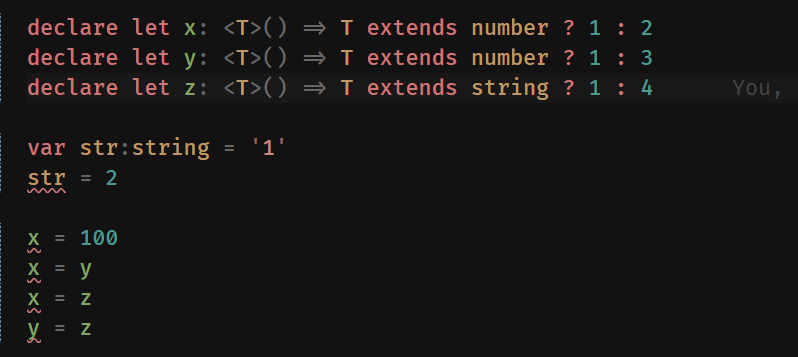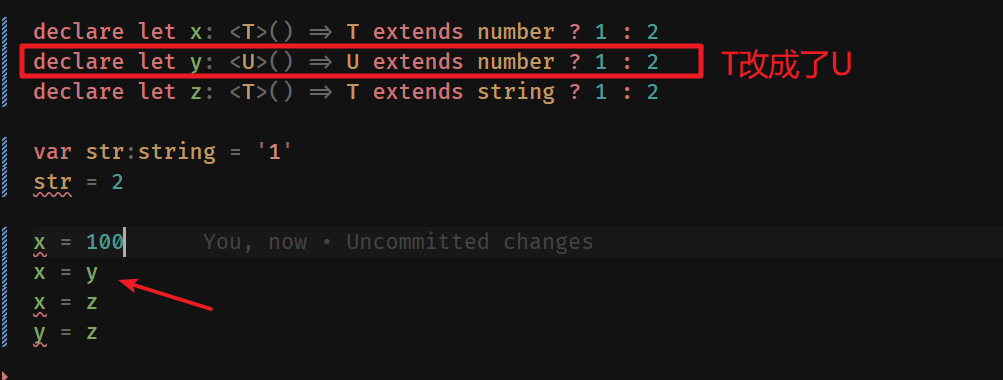# TS类型体操 之 extends,Equal,Alike 使用场景和实现对比

7/4/2022 TS

## # extends 的作用

``````
// 🌰 - 1
type ID = string | number
type TestID = ID extends string ? true : false  // false
type TestID2 = string extends ID ? true : false // true

// 🌰 - 2
type UnionType = string | number | boolean
type testUnion = string extends UnionType ? true : false // true
type testUnion2 = [string] extends [UnionType] ? true : false // true
type testUnion3 = [UnionType] extends [string] ? true : false // false
``````
1
2
3
4
5
6
7
8
9
10
11
12

ID 是 string/number 类型，所以 ID 取值的范围是比 string 类型的范围的大的，TestID 返回值自然为 false 了

### # 用 extends 的特性解决 01097-medium-isunion

extends 是一个自带范围判断的判断符，只要在另外一个类型范围内，那就判定为 true

01097-medium-isunion 题目的需求：判断一个传入的类型是否 union 类型

``````type cases = [
Expect<Equal<IsUnion<string>, false >>,
Expect<Equal<IsUnion<string|number>, true >>,
Expect<Equal<IsUnion<'a'|'b'|'c'|'d'>, true >>,
Expect<Equal<IsUnion<undefined|null|void|''>, true >>,
Expect<Equal<IsUnion<{ a: string }|{ a: number }>, true >>,
Expect<Equal<IsUnion<{ a: string|number }>, false >>,
Expect<Equal<IsUnion<[string|number]>, false >>,
// Cases where T resolves to a non-union type.
Expect<Equal<IsUnion<string|never>, false >>,
Expect<Equal<IsUnion<string|unknown>, false >>,
Expect<Equal<IsUnion<string|any>, false >>,
Expect<Equal<IsUnion<string|'a'>, false >>,
]
``````
1
2
3
4
5
6
7
8
9
10
11
12
13
14

• `string extends string | number` 为 true
• `string | number extends string` 为 false
• TS 体操中的 Union（联合类型）会自动 “解构”

``````type IsUnion<T,U = T> = T extends U ? U extends T ? false : true : false
``````
1

`IsUnion` 里面，T 会自动的 “解构”，就比如 传入的是 `string|number`

1. T 会自动变成 string，然后和 U 进行比较
2. T 在变成 number ，在和 U 比较
3. U 同理 变成 string 和 T（string/number） 进行比较

``````type TestUnion<T extends string, U extends string = T> = `\${T}-\${U}`
type ResultUnio = TestUnion<'a' | 'b'>

// type ResultUnio = "a-a" | "a-b" | "b-a" | "b-b"
``````
1
2
3
4

• `{ a: string|number }``[string|number]` 不是联合类型，因为他们其实都属于同一个对象下的，他们的属性值是联合类型，而他们自身并不是

• 剩下的最后 4 个测试用例中，比如 `string|never` , `string|'a'` 也是不符合 多个类型联合 的意义

• never 说明没有一个类型符合，就好像绝育的小猫咪和正常的小猫咪还能生出新的小猫咪吗？不行的嘛。所以他们不能联合
• 至于 `string|'a'` 'a' 本来就是属于 string 类型的，这就好像 一只公的小猫和一只公的黑色小猫，他们能 联合 吗？他们都属于 `公猫`，只有并集，联合不出来结果
• 所以 `string|any` 同上面同理，any 包罗一切，没有东西可以和 any 组成联合

TS 会自动帮他们取范围比较大的一个类型作为联合结果

``````type StringUnion = string | 'a' // 结果为 string
type StringUnion2 = string | any // 结果为 any
``````
1
2

## # 使用小技巧实现 Equal 全等判断

``````type User = {
name?: string
age: number
}

type User2 = {
name?: string
} & {
age: number
}

type R = Equal<User1, User2> // false
type R2 = User1 extends User2 ? (User2 extends User1 ? true : false) : false // true
``````
1
2
3
4
5
6
7
8
9
10
11
12
13
14
15

``````export type Equal<X, Y> =
(<T>() => T extends X ? 1 : 2) extends
(<T>() => T extends Y ? 1 : 2) ? true : false
``````
1
2
3

• T 是那里冒出来的？
• T 是作为泛型，传入一个函数内，然后在返回出来？
• T 为什么会等于 X ？
• T 又为什么等于 Y 了 ？

• `(<T>() => T extends X ? 1 : 2)` 假设为 1 式
• `(<T>() => T extends Y ? 1 : 2)` 假设为 2 式

`<T>()` 怎么理解呢？有一句很重要的话 (也是摘自 stackoverflow 上的答案)

The assignability rule for conditional types <...> requires that the types after extends be "identical" as that is defined by the checkerF

``````declare let x: <T>() => T extends number ? 1 : 2
declare let y: <T>() => T extends number ? 1 : 2
declare let z: <T>() => T extends string ? 1 : 2

var str: string = '1'
str = 2 // 报错

x = 100 // 报错
x = y
x = z // 报错
y = z // 报错
``````
1
2
3
4
5
6
7
8
9
10
11
• 2 赋值给 str 时会报错 Type 'number' is not assignable to type 'string'.
• x 赋值为 100 时会报错 Type 'number' is not assignable to type '<T>() => T extends number ? 1 : 2'.

• y 赋值给 x 啥事都没有

• z 赋值给 x 和 z 赋值给 y 都会提示下面的错误

``````Type '<T>() => T extends string ? 1 : 2' is not assignable to type '\<T\>() => T extends number ? 1 : 2'.
Type 'T extends string ? 1 : 2' is not assignable to type 'T extends number ? 1 : 2'.
Type '1 | 2' is not assignable to type 'T extends number ? 1 : 2'.
Type '1' is not assignable to type 'T extends number ? 1 : 2'.
``````
1
2
3
4## # 和 Equal 很像的 Alike

Alike 和 Equal 一样是为了判断相等的，在之前一篇文章 《TS 类型体操 之 循环中的键值判断,as 关键字使用》 中有讲过的测试用例就是用的 Alike

``````type User = {
name?: string
age: number
}

type User2 = {
name?: string
} & {
age: number
}

// 把 Equal 换成 Alike 得到的就是 true 的结果
type R = Alike<User1, User2> // true
type R2 = User1 extends User2 ? (User2 extends User1 ? true : false) : false // true
``````
1
2
3
4
5
6
7
8
9
10
11
12
13
14
15
16

Alike 不能就单纯的理解是就是 T extends U && U extends 的实现，绝对不能

• Alike 实现如下：
``````export type MergeInsertions<T> = T extends object ? { [K in keyof T]: MergeInsertions<T[K]> } : T

export type Alike<X, Y> = Equal<MergeInsertions<X>, MergeInsertions<Y>>
``````
1
2
3

`{} & {}` 经过 MergeInsertions 处理后，也会被合并为一个对象 `{}`，然后在拿去 Equal 对比。不得不说，真是妙

``````type TestUnion = { a: string } | { a: number }

type IsLike = Alike<TestUnion, TestUnion> // true
type IsUnionResult = IsUnion<TestUnion> // true

type IsLike2 = Alike<string, string> // true
type IsUnionResult2 = IsUnion<string, string> // false
``````
1
2
3
4
5
6
7

## # 总结

• extends 是一个 子集的比较，只要 x 是 y 的子集，那么 x extends y 就为 true
• 判断一个类型是否 union 类型，用到的也是 extends 子集 的特点
• 如果 x 是 y 的子集，那么 y 就不可能是 x 的子集
• x extend y 并且 y 又 extends x 的话，那只能说他们都是 单一的类型，不存在联合
• Equal 函数很巧妙的用了 TS 的一个特性
• `The assignability rule for conditional types <...> requires that the types after extends be "identical" as that is defined by the checkerF`
• 通过一个类似公式代入的场景判断 2 个类型是否全等
• 单一类型 和 交叉类型 `不全等`
• 如果想把条件放宽松点，只想判断 2 个类型所有的字段都相同就是相等的话
• 就要用上 Alike ，Alike 则是用了一个 `MergeInsertions`(Clone 升级版) 来把交叉类型合并为 单一类型
• 有了单一类型，就可以用 Equal 来对比了
• 对于单一类型，T extends U && U extends 可以判断出是否 Union ，而 Alike 只能判断他们是否相等（所以不要搞混 Alike 和 IsUnion 的实现原理）

Last Updated: 7/5/2022, 12:29:28 AM